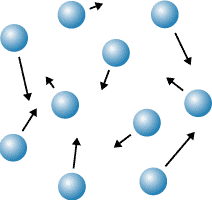Examples & Random Walks in 2D and 3D

What does a random walk have to do with real life?

Whew! Now that we're through with all of that math, how can we relate random walks to real life? Random walks and the mathematics that govern them are found everywhere in nature. When gas particles bounce around in a room, changing direction every time they collide with a another particle, it is random walk mathematics that determines how long it will take them to travel from one location to another. The particles in a drop of food coloring added to a glass of water will spread out, partially due to currents in the water, and partially due to a random walk.Random walks and baseball????

Random walks can even apply to baseball. Consider a baseball team that is perfectly average -- that is, they have a 50% chance of winning or losing each game. The outcome of each game can be +1 (if they win) or -1 (if they lose). Then as the baseball season progresses, this perfectly average team hops forward and backward on the number line. Their position "d" on the number line indicates how many more games they have won than lost (a negative "d" means that they have lost more games than won). There are 162 games in a baseball season, so for a perfectly average team, we would expect sqrt(162) (approximately 12-13) more games won than lost or more games lost than won to be completely normal. A difference of 12 would be a record of 87-75 or 75-87. Sometimes a single game will determine who advances to the playoffs, but roughly 12 games out of every season are completely determined by chance!

What about the world series? The world series is only 7 games long. For two equally matched teams (50% probability of either team winning), we would expect the number of wins minus the number of losses to be roughly sqrt(7) or 2.6. So roughly 2-3 games out of 7 are determined completely by chance. No wonder it's so hard to predict the winner!

Random walks in more than one dimension

Of course the 1-dimensional random walk is easy to understand, but not as commonly found in nature as the 2D and 3D random walk, in which an object is free to move along a 2D plane or a 3D space instead of a 1D line (think of gas particles bouncing around in a room, able to move in 3D). We won't work out the math here, but it turns out to be governed by the same rule as a 1D random walk -- the total distance traveled from where it started is approximately sqrt(N) where N is the number of steps taken.

Random walks with a variable step size

In the derivation on the previous page, we assumed a dimensionless step size of 1. In real life, step sizes are measured in units of distance and sqrt(<d2>)=<r>sqrt(N), where "r" is the size of a step, measured in units of length, and <r> is the average size of a step if the random steps vary in size (which they typically do in nature).

Now that we know a little more about random walks, let's take another look at the video we saw before. The video shows the paths of 7 black dots undergoing a random walk in 2D. All of the dots start out in the same place and then start to wander around. Notice that the steps they take are not all the same length. By the end of the video, some of the dots ended up above where they started, some below, some to the right, and some to the left. Some don't end up very far from where they started and others manage to travel quite a distance.Not all random walks are "random"

So far all of the random walks we have considered allowed an object to move with equal probability in any direction. But not all random walks follow this rule. A biased random walk is a random walk that is biased in one direction, leading to a net drift on average of particles in one specific direction.

There are several ways that a random walk can be biased. Think back to our 1D number line.

• Suppose that instead of an equal probability of moving left to right, there was a higher probability of moving to the right.
• Or, suppose the probabilities of moving to the left or right remained equal, but whenever the dot moved to the right, it moved 2 spaces, and when it moved to the left, it only moved 1 space.

In both of these cases, we would expect a net drift to the right. In other words, the random walk is biased toward the right. The video below shows a biased random walk in 2D. Can you guess which of the two mechanisms listed above is responsible for the bias?If you guessed the second mechanism, you're right! Each black dot has equal probability of stepping towards the right or towards the left. But the step size is on average longer if the step is towards the right than if the step is towards the left.

Photo credit: (food coloring) Jovanna Lopez, http://www.soasoas.com/april/gallery/viewImg2.cgi?dir=stillLife&id=Swirls_of_Color